Study Materials

# NCERT Solutions for Class 9th Mathematics

Page 1 of 2

## Chapter 12. Herons Formula

### Exercise 12.1

Exercise 12.1

Q1. A traffic signal board, indicating ‘SCHOOL AHEAD’, is an equilateral triangle with side ‘a’. Find the area of the signal board, using Heron’s formula. If its perimeter is 180 cm, what will be the area of the signal board?

Solution:Perimeter of traffic signal board = 180 cm

Side of traffic signal board

Using equation (1), area of traffic signal board

Q2:The triangular side walls of a flyover have been used for advertisements.The sides of the walls are 122 m, 22 m and 120 m (see Fig. 12.9). The advertisements yield an earning of Rs 5000 per m2 per year. A company hired one of its walls for 3 months. How much rent did it pay?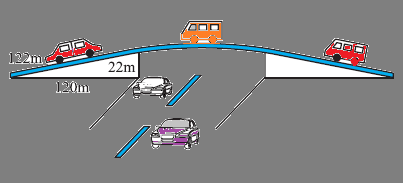Solution:

The sides of the triangle a,b,c are of 122 m, 22 m, and 120 m respectively.

Perimeter of triangle = (122 + 22 + 120) m

2s = 264 m

s = 132 m

By Heron's formula,

Rent of 1 m2 area per year = Rs 5000

Rent of 1 m2 area per month = Rs

Rent of 1320 m2 area for 3 months =

= Rs (5000 × 330) = Rs 1650000

Therefore, the company had to pay Rs 1650000

Q3: There is a slide in the park. One of its side walls has been painted in the same color with a message “KEEP THE PARK GREEN AND CLEAN” (see the given figure). If the sides of the wall are 15m, 11m, and 6m, find the area painted in color.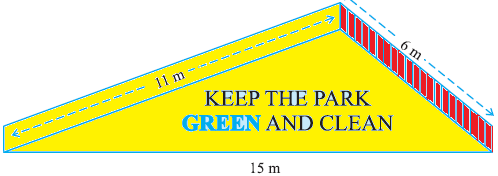It can be observed that the area to be painted in colour is a triangle, having its sides as 11 m, 6 m, and 15 m.

Perimeter of such a triangle = (11 + 6 + 15) m

s = 32 m

s = 16 m

By Heron's formula

Area of triangle=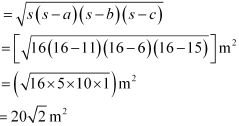Therefore, the area painted in color is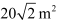Q4 :  Find the area of a triangle two sides of which are 18 cm and 10 cm              and the perimeter is 42 cm.

Let the third side of the triangle be x.

Perimeter of the given triangle = 42 cm

18 cm + 10 cm + c = 42

c = 14 cm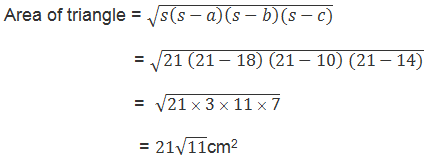5. Sides of a triangle are in the ratio of 12: 17: 25 and its perimeter is 540cm. Find its area of triangle.

Solution:

: Let the common ratio between the sides of the given triangle be x.

Therefore, the side of the triangle will be 12x, 17x, and 25x.

Perimeter of this triangle = 540 cm

12x + 17x + 25x = 540 cm

54x = 540 cm

x = 10 cm

Sides of the triangle will be 120 cm, 170 cm, and 250 cm.Q6 :  An isosceles triangle has perimeter 30 cm and each of the equal                sides is 12 cm. Find the area of the triangle.

Solution:

Let the third side of this triangle be x.

Perimeter of triangle = 30 cm

12 cm + 12 cm + x = 30 cm

x = 6 cm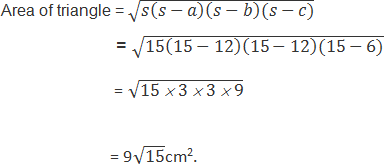Page 1 of 2

Chapter Contents: